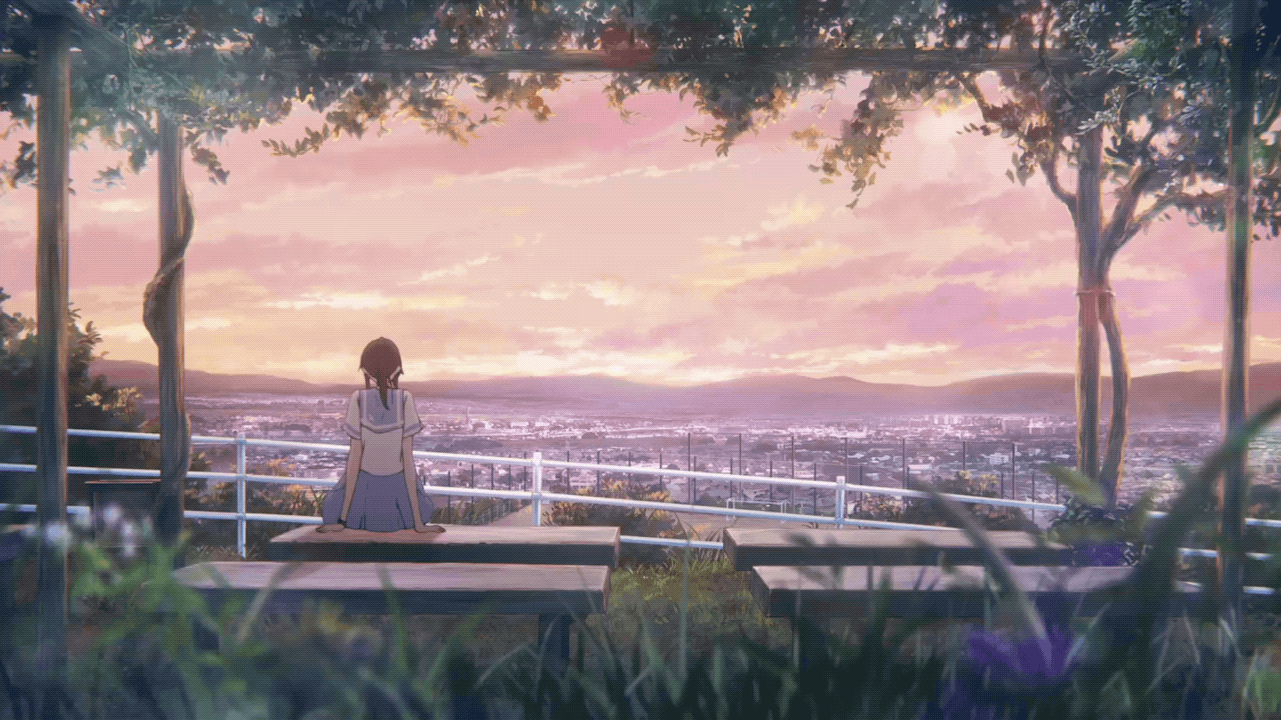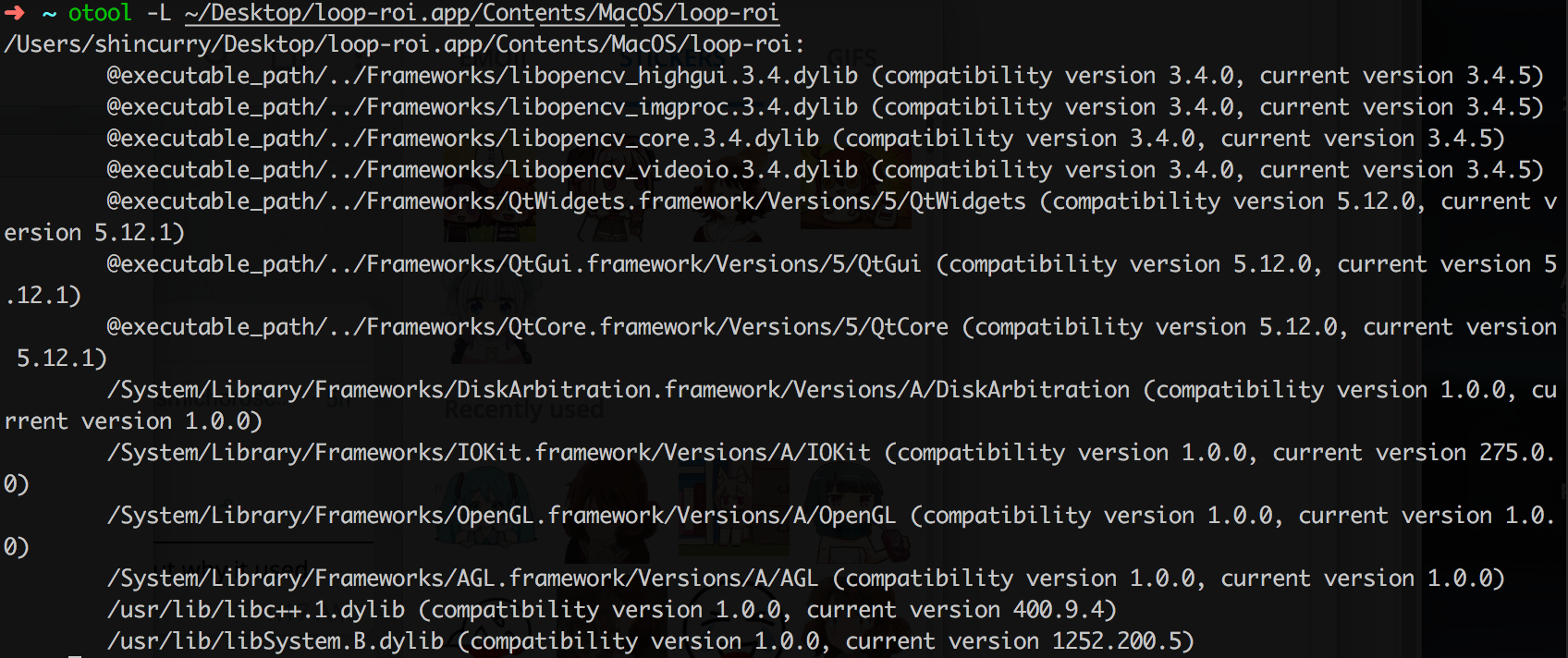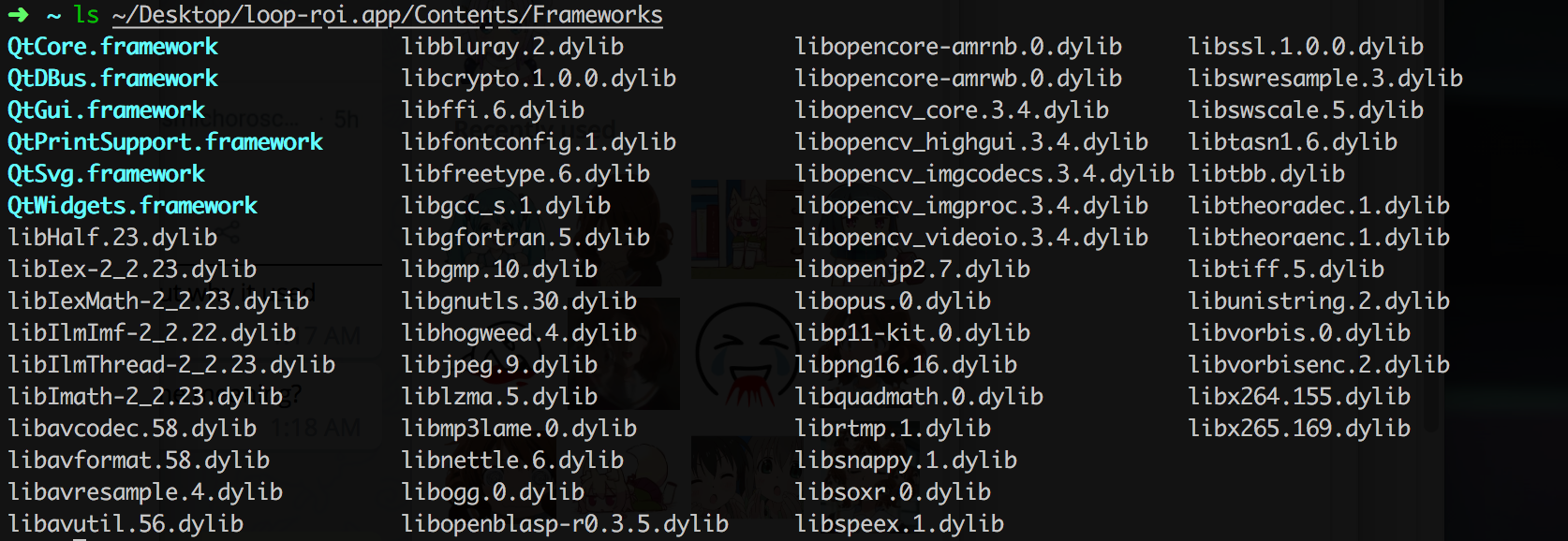# 短视频局部区域静态化

OpenCV Mar 16, 2019

.-..-..-.

``````ffmpeg -i input -i logo -filter_complex 'overlay=10:main_h-overlay_h-10' output
``````

https://github.com/moeoverflow/loop-roi

PS: loop-roi 这个名字只是一个临时的名字，表示这个项目主要的功能就是做循环视频的区域静态化。实际上以后还做了其他一些处理视频的小项目，可能就会把这些小项目全部合进一个主要的软件项目了。当然这是以后的事情了。

loop-roi 的使用方法很简单，打开一个视频文件（短视频，只有几秒的那种），然后在画面中用鼠标选中需要静态化的区域，如果觉得效果满意导出就行。

1. 加载视频并在界面中循环播放
2. 捕获鼠标选中的区域的像素点坐标
3. 计算出所有包含在选中区域内的像素点
4. 替换视频中每一帧区域内的像素值
5. 导出结果到视频文件

``````virtual void mouseMoveEvent(QMouseEvent *event)
virtual void mousePressEvent(QMouseEvent *event)
virtual void mouseReleaseEvent(QMouseEvent *event)
``````https://en.wikipedia.org/wiki/Point_in_polygon

``````// based on https://stackoverflow.com/a/15599478
bool LoopRoi::pointInPolygon(QPoint point, QVector<QPoint> polygon) {
int i, j, nvert = polygon.size();
if (nvert == 0) return false;
bool c = false;

QPoint * points = polygon.data();
for(i = 0, j = nvert - 1; i < nvert; j = i++) {
if( ( (points[i].y() >= point.y() ) != (points[j].y() >= point.y()) ) &&
(point.x() <= (points[j].x() - points[i].x()) * (point.y() - points[i].y()) / (points[j].y() - points[i].y()) + points[i].x())
)
c = !c;
}

return c;
}
````````````#### import all opencv components ####
QT_CONFIG -= no-pkg-config
PKGCONFIG += opencv

macx {
PKG_CONFIG = /usr/local/bin/pkg-config
}

# ----------------------------

#### import specific opencv components ####
macx {
INCLUDEPATH += /usr/local/include
LIBS += `/usr/local/bin/pkg-config opencv --libs-only-L` -lopencv_core -lopencv_imgproc -lopencv_highgui -lopencv_videoio
unix:QMAKE_RPATHDIR += @executable_path/../Frameworks
}

``````